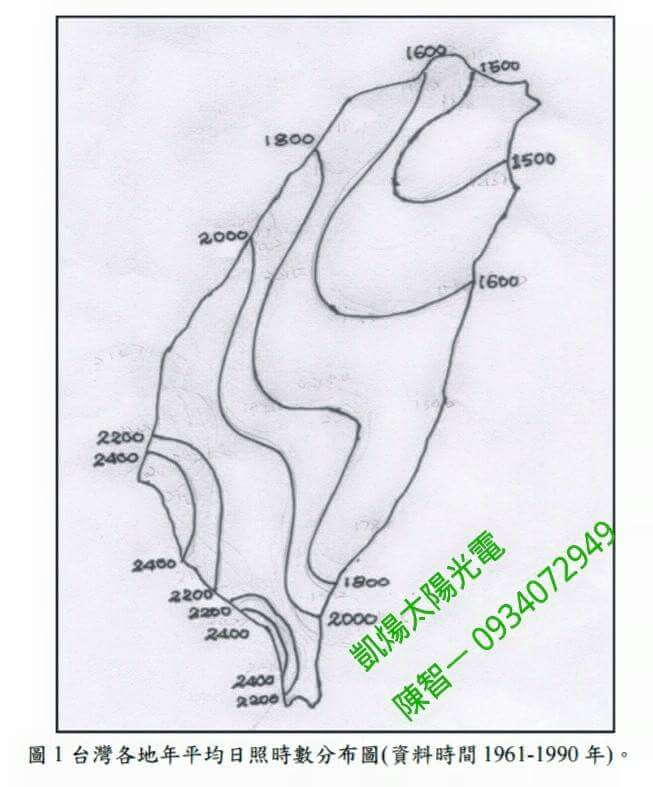# 你該知道的太陽能電廠投資(一)：法令保障與收益計算## 太陽能電廠年收入的計算其實就是當地每千瓦年發電量×裝置容量

10*3.51*365*6.6721≈85,000

50*3.51*365*5.5760≈357,000

1.如果非設備貸款則不強制保險
2.設備結構保險公司認定不夠安全不承保

85,000×20=1,700,000

170,000

58,800×15=882,000

85,000×5%×15=63,750

85,000×5%×10=42,500

1,700,000 – 882,000 – 63,750 – 42,500 – 170,000 = 541,750

(27,088÷170,000)×100%= 16%
(你問我為什麼是用17萬而不是用85萬去除，對啊為什麼呢……)

357,000×20=7,140,000

760,000

262,947×15=3,944,218

357,000×5%×15=267,750

357,000×5%×10=178,500

7,140,000 – 3,944,218 – 267,750 – 178,500 – 760,000 = 1,989,532

(99,477÷760,000)×100%= 13%
(你又問我為什麼是用76萬而不是用380萬去除，噯呀你怎麼又問呢……)「南部多於北部，海邊多於山邊」